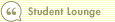Course 403: Introduction to Discounted Cash Flow
Cost of CapitalIn this course1 Introduction 2 Estimating Future Cash Flow 3 Discounting and Discount Rates 4 Cost of Capital 5 Two Types of Capital, Two Costs 6 The Perpetuity Value 7 The Bottom LineThe rate we use to discount a company's future cash flows back to the present is known as the company's required return, or cost of capital.

A company's cost of capital is exactly as its name implies. When a company raises capital from its lenders and owners, both types of investors require a return on their investment. Lenders expect to be paid interest on their loans, while owners expect a return, too.

A stable, predictable company will have a low cost of capital, while a risky company with unpredictable cash flows will have a higher cost of capital. That means, all else equal, that the riskier company's future cash flows are worth less in present value terms, which is why stocks of stable companies often look more expensive on the surface. The cost of capital used in a DCF model can have a significant impact on the fair value, so it's important to pay attention to this estimated figure.

The rate you would use to discount cash flows if using the "cash flow to the firm" method is actually a company's weighted average cost of capital, or WACC. A company's WACC accounts for both the firm's cost of equity and its cost of debt, weighted according to the proportions of equity and debt in the company's capital structure. Here's the basic formula for WACC:

(Weight of Debt)(Cost of Debt) + (Weight of Equity)(Cost of Equity)

For example, if the market value of a company's equity is \$600 million and it has \$400 million of debt on its balance sheet, then 60% of its capital is equity and 40% is debt. If the company's cost of equity is 10% and its cost of debt is 7%, then its WACC is:

(60% x 10%) + (40% x 7%) = 8.8%

Note: If using the "cash flow to the firm" DCF method, the WACC would be your discount rate. However, this method does not discount free cash flow. Rather, it discounts operating earnings before interest but after taxes. Arriving at this figure involves complicated adjustments for interest and taxes. To keep it simple, we will just use the "free cash flow to equity" method in our example in the next lesson.Search Print Lesson  | FeedbackDel.icio.us |digg itLearn how to invest like a pro with Morningstar’s Investment Workbooks (John Wiley & Sons, 2004, 2005), available at online bookstores.© Copyright 2015 Morningstar, Inc. All rights reserved. Please read our Privacy Policy. If you have questions or comments please contact Morningstar.